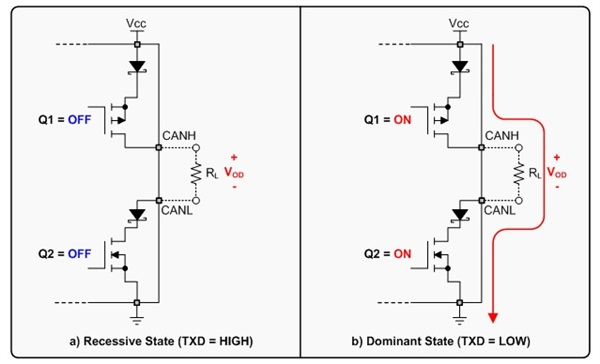# CAN BUS electrical characteristics

I know that CAN bus has two states. Dominant states where CANH goes 3.5V and CANL goes to 1.5V and in recessive state, both CANH and CANL goes to 2.5V. My question is, how much current does it draw in dominant and recessive states?

You may(1) find the information in the datasheet.
Some give the information directly, for example the TCAN330 from TIOther datasheet give it indirectly, for example the SN65HVD23x from TIHere, the current required for the IC itself is given by $$\I_{CC}\$$ Supply current. Note the "No load" condition.
Typically, the bus load is 60 Ω.
In dominant state the Differential output voltage in dominant state $$\V_{OD(D)}\$$ is typically 2V and max 3V.
So, with a bus load of 60 Ω, there is a typical current drawn of 2V/60 Ω = 33 mA, and max 50 mA.

(1) Unfortunately, not all datasheet are well documented.

According to this article from Texas Instruments, a typical CAN bus driver output stage will look something like this:Thus, we can expect the bus to draw significantly more current in the dominant state. Unless we know the termination (load) resistance, differential voltage (CANH-CANL), and maximum output current of the drive IC, we can't determine the exact current in the dominant state.

In the recessive state, the current will be nearly zero since nothing is actively driving the bus.

the receiver interface circuits will be monitoring the bus, either to detect collisions during transmitting, or for external data packets; this requires an analog comparator with about 20 nanosecond delay time, with stable hysteresis, and with input voltage-dividers on both of Vin+ and Vin- pins of the comparator, to level shift DOWN into 0/5volt rails from a CAN bus that during one-sided failures (Bus shorts) can be up to 40 volts.

For 10 nanosecond delay time, allowing another 10nanoseconds for the analog comparator, and assuming 10pF ESD and analog-differential-pair gate-capacitrances, you need 1Kohm resistance in the input voltage-divider, with 9Kohm also used.